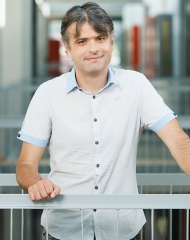• Course code:63532
• Credits:6
• Semester: summer
• Contents

Do not be scared by the mathematics word in the title. Discrete mathematics is the region of mathematics planet that works best with computers. In mathematics we are often satisfied with a single solution to the problem, in computer science this is almost never the case. Among all possible solutions we shall look for the one that can be rewritten as an efficient algorithm (or, not equivalent, can be efficiently rewritten as an algorithm).

Mostly we shall work on problems in graph theory. Let me pin out a pair of problems that we shall keep running into: graph coloring and the problem of disjoint paths. In general, graph coloring problems are hard (in the theoretical sense). Yet if we only consider a subclass of graphs, planar graphs for example, even graph coloring problems become easy enough to work on. The problem of disjoint paths can be generalized into several directions, directed or undirected graphs, vertex- or edge- disjoint, separate terminals or not, looking for bottlenecks, maximizing flows.

Most of the problems we shall work with will become easy on a restricted set of graphs having the property, that a small collection of cops can catch a fast robber, which will lead us to the land of graph decompositions.

Finally we shall dig into a collection of problems from computational geometry: segment intersections, polygon triangulations, Voronoi diagrams and Delaunay triangulations.

The students are required to have sufficient knowledge in the area of algorithms and data structures and have a command on time and space complexity of algorithms.

Student work includes weekly homework assignments and a final exam.

• Study programmes
• Distribution of hours per semester
45
hours
lectures
30
hours
laboratory work
• ProfessorTeaching Assistant
Room:R3.23-LMMRI